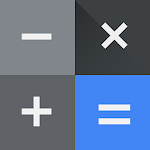• Author:

• Version:

8.1 (403424005)

• Update on:

## The description of Calculator

Calculator provides simple and advanced mathematical functions in a beautifully designed app.

• Perform basic calculations such as addition, subtraction, multiplication, and division
• Do scientific operations such as trigonometric, logarithmic, and exponential functions